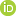Title Short-term load forecasting using the combined method of wavelet transform and neural networks tuned by the Gray Wolf optimization algorithm Tweet Mohammadali Zanjani, S; Shahinzadeh, H; Moradi, J; Fayaz-Dastgerdi, M -H; Yaici, W; Benbouzid, M 2022 IEEE Global Energy Conference (GEC2022); 2022 p. 1-6, https://doi.org/10.1109/GEC55014.2022.99867112022 Natural Resources Canada, Contribution Series 20230021 IEEE Global Energy Conference; Batman; TR; October 22-29, 2022 serial English paper; digital; on-line pdf Science and Technology; Artificial intelligence; Distribution; Forecasting figures; flow charts; graphs CanmetENERGY - Ottawa Buildings and Renewables Group - Hybrid Energy Systems 2022 10 26 Daily load forecasting in distribution companies, which is to present to the network management company and adopt the appropriate load management method, is considered an inseparable matter in distribution networks. Accurate load forecasting will reduce the price of electricity generation in the power network. In the discussion of exploitation and capability, The reliability of the power network is effective and necessary. Therefore, it is difficult to accurately predict the load due to the influence of load patterns on various factors such as weather, economic, and social factors. For this reason, they have addressed load forecasting problems in recent years using different methods. In this article, the goal is to achieve a combination of Neural Network-Wavelet Transform that can accurately predict the load of distribution networks. The data used in this model is electric charge. Wavelet Transform has been used for multimodal forecasting, filter type, window length (the number of past data used in forecasting), and the number of hidden layers for each neural network has been optimized by the gray wolf algorithm to minimize the forecasting error. Also, the data used in the analysis, evaluation, and short-term forecasting is based on the actual load data of the electricity distribution network of Isfahan province. (Plain Language Summary, not published)Daily load forecasting in distribution companies, which is to present to the network management company and adopt the appropriate load management method, is considered an inseparable matter in distribution networks. Accurate load forecasting will reduce the price of electricity generation in the power network. In the discussion of exploitation and capability, the reliability of the power network is effective and necessary. Therefore, it is difficult to accurately predict the load due to the influence of load patterns on various factors such as weather, economic, and social factors. For this reason, in recent years, load forecasting problems have been addressed using different methods. In this article, the goal is to achieve a combination of Neural Network-Wavelet Transform that can accurately predict the load of distribution networks. The data used in this model is electric charge. Wavelet Transform has been used for multimodal forecasting, filter type, window length (the number of past data used in forecasting), and the number of hidden layers for each neural network has been optimized by the Grey Wolf algorithm to minimize the forecasting error. Also, the data used in the analysis, evaluation, and short-term forecasting is based on the actual load data of a province electricity distribution network. 331838